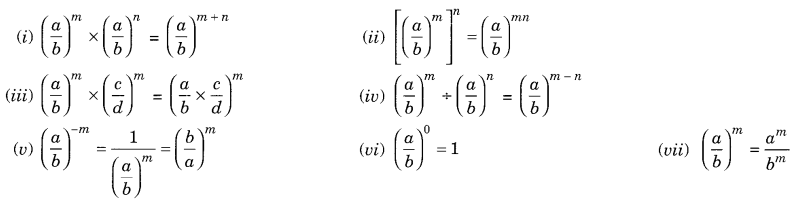On this page, you will find Exponents and Powers Class 8 Notes Maths Chapter 12 Pdf free download. CBSE NCERT Class 8 Maths Notes Chapter 12 Exponents and Powers will seemingly help them to revise the important concepts in less time.

## CBSE Class 8 Maths Chapter 12 Notes Exponents and Powers

### Exponents and Powers Class 8 Notes Conceptual Facts

• A numeral (a)n is called an exponential expression where a is called base and n the exponent or power.
an = a x a x a x a……… x a (n times)
• A negative rational number raised to an even power is always positive, $$\text { e.g. },\left(-\frac{1}{2}\right)^{4}=\frac{1}{16}$$
• A negative rational number raised to an odd power is always negative, i.e., $$=\left(-\frac{1}{2}\right)^{3}=-\frac{1}{8}$$

Laws of exponents: